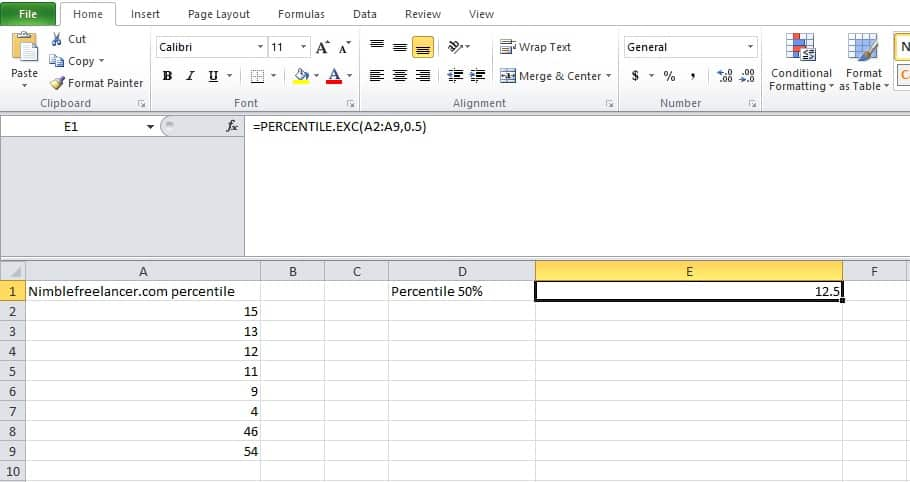# How to Calculate Percentile in Excel?Microsoft Excel is an indispensable tool for any entrepreneur or a small-scale business owner. It is essential for executing the necessary calculations for your business. Out of all, the excel function which is extremely useful is the PERCENTILE.EXC function. PERCENTILE.EXC function computes the “kth percentile” for a data set where k is 0 to 1.

## What is a percentile?

A percentile represents a number where a certain percentage of scores falls below that number. For example, if you are the fifth tallest person in a group of 20, 75% of people are shorter than you, so you are at the 75th percentile.

In other words, PERCENTILE.EXC function will skim through a vast data set and pinpoint the exact number, which helps segregate the data into your chosen percentiles. On the other hand, Microsoft Excel also includes PERCENTILE.INC function. Although it is less accurate, it comes in handy in many situations.

You can learn percentile calculation and much more in the free Exploring and Producing Data for Business Decision Making course, where all students will learn how to calculate percentile and Z scores.

## Calculate percentile in Excel## How to Calculate Percentile in Excel?

To find percentile in Excel, you need to enter a formula into cell “=PERCENTILE. EXC(A1:AX,k)” without quotes.

“X” represents the last row in column “A,” where you have entered data

“k” represents the percentile value from 0 to 1.

The k percentile represents a value below which k percent of values in the data set fall.

A percentile calculated with k = 0.5 means 50% percent of values are less than or equal to the calculated result.

## How to Find Percentile in Excel?

• Open a new worksheet in Microsoft Excel.
• Go ahead and click on cell “A1”. Then, enter the values into the cells in column A.
•  Click the “B1” cell.
• Enter =PERCENTILE.EXC(A1: AX,k) into the cell. Here, “X” denotes the last row in column “A.” So you will have to enter the data in column “A.”And “k” is the percentile value you are looking for. The percentile value for “k” must range between  0-1.Example: If you want to find the value for the 60th percentile, you will use “0.6” as your percentile value.
•  Click “Enter” to complete your formula. Lastly, check the value in “B1.”

## How to calculate p50 p75 p90 in Excel?

To calculate p50, you need to use the “=PERCENTILE. EXC(A1:AX,k)” formula where “X” is the last row in column “A” where you have entered data and “k” is 0.5.
To calculate p75, you need to use the “=PERCENTILE. EXC(A1:AX,k)” formula where “X” is the last row in column “A” where you have entered data and “k” is 0.75.
To calculate p90, you need to use the “=PERCENTILE. EXC(A1:AX,k)” formula where “X” is the last row in column “A” where you have entered data, and “k” is 0.9.